# Introduction

mWaveD is an R-package that allows the user to deconvolve a set of signals that contain a common function of interest. This package extends and generalises the work previously done in the waved package available at (Raimondo and Stewart 2012) and discussed in (Raimondo and Stewart 2007). The waved method is based on the results of (Johnstone et al. 2004). Similar to waved, the estimation framework is implemented in the Fourier domain and utilises the Fast Fourier Transform (FFT). However, instead of using the base R FFT subroutines, mwaved implements instead the Fastest Fourier Transform in the West (FFTW) subroutines written in C introduced in (Frigo and Johnson 2005). This is implemented via the Rcpp package and achieves much faster calculations than the waved package. This document is broken into the following structure:

## Background and context

The goal of the package is to allow the user to estimate some periodic function of interest $$f$$ when they only have access to a set of noisy and convolved (blurred) data of the form, $Y_{i,\ell} = g_{\ell,n} \star f_n[i] + e_{i,\ell}; \quad i = 1, 2, \ldots, n; \quad \ell = 1, 2, \ldots, m,$ where $$f_n[i] = f(x_i)$$ with $$x_i = \tfrac{i-1}{n}$$ for $$i = 1, 2, \ldots, n$$ and $$f : (0,1) \to \mathbb{R}$$ is a (0,1) periodic function and $$f_n[i]$$ denotes the discrete realisation available in the data. Define $$g_{\ell,n}[i]$$ similarly to $$f_n[i]$$ via a suitable function, $$g_\ell$$. The circular convolution operator is then denoted $$k \star f_n[i]$$ and defined, $k \star f_n[i] = \sum_{j = 1}^n f_n[j]k[i-j],$ and $$e_{i,\ell}$$ denote a set of error variables with mean zero that are possibly long-range dependent. Long-range dependence does not have a universal definition, however in this case, assume the variables $$e_{i,\ell}$$ have the following covariance structure, $$\mathbb{E} e_{1,\ell} e_{j +1, \ell} \sim C j^{-\alpha_\ell}$$ for $$\alpha_\ell \in (0,1).$$ Then by appealing to the results in (Wang 1996), (Wang 1997) and (Taqqu 1975); for $$t \in (0,1)$$, the cumulative process, $Cn^{\alpha_\ell/2 - 1}\sum_{j = 1}^{\lfloor nt \rfloor} e_{j,\ell} \stackrel{p}{\longrightarrow} B_{H_\ell}(t).$ The interested reader is referred to (Wishart 2013), (Wang 1996) and (Wang 1997) for details.

The previous result motivates the following asymptotic model, $dY_\ell(x) = f*g_\ell(x) \, dx + \varepsilon^{\alpha_\ell} dB_{H_\ell}(x), \quad \ell = 1, 2, \ldots, m.$ Here, $$f$$ is a (0,1) periodic function and $$f*g$$ denotes the integral convolution operator, $f*g(x) = \int_0^1 f(t)g(x-t)\, dt$ and $$B_{H_\ell}(x)$$ denotes a fractional Brownian motion. This asymptotic model was investigated in (Kulik, Sapatinas, and Wishart 2014) and upper bounds on the $$\mathscr L_p$$ risk established ($$1 \le p \le \infty$$). The mwaved package is based on those results. In that paper an asymptotic model is considered and a link and justification for the results in the finite model presented here. The interested reader is referred to there for more details.

The original WaveD transform was developed in (Johnstone et al. 2004) and refined in subsequent publications before it became widely available in the waved R package discussed in (Raimondo and Stewart 2007). Since that time, theoretical extensions have been made to a near functional data setting where multiple channels of data are available considered in (Pensky and Sapatinas 2009), (Petsa and Sapatinas 2009), (Pensky and Sapatinas 2010) and (Pensky and Sapatinas 2011) among others. There has also been an investigation into the box car class of convolving functions that have received attention in (Kerkyacharian, Picard, and Raimondo 2007), (Pensky and Sapatinas 2011), (Benhaddou et al. 2014) and others. This package uses the same waved type paradigm for estimation but applies it to the more general context given the recent extensions in the literature. As alluded to earlier, it pays particular attention to the formulation and results given in (Kulik, Sapatinas, and Wishart 2014).

On top of the faster numerical implementation using FFTW, the extensions and generalisations that mwaved uses over the waved package are the following points.

• Multichannel: Analysis of multiple channels of data instead of only a single channel (i.e. waved considers the case $$m = 1$$, while mwaved allows $$m > 1$$)
• Convolving functions: Between each channel, the convolving functions are members of the same class but of possibly different severity. That is, there is a set of convolving functions $$\left\{ g_\ell \right\}_{\ell = 1}^m$$ are assumed to belong to one of three different classes defined in the Fourier domain where $$g_{\omega,\ell} = \int_\mathbb{R} g_\ell(x) e^{-2\pi i \omega x}\, dx$$ denotes the Fourier transform of $$g_\ell$$. Numerically, the user only has knowledge of $$g_\ell$$ on a finite grid of points denoted $$g_\ell[i]$$ for $$i = 1, 2, \ldots, n$$. These three classes are defined in the Fourier domain are:
• (regular) ‘smooth’ class where $c_\ell|\omega|^{-\nu_\ell} \le |g_{\omega, \ell}| \le C_\ell|\omega|^{-\nu_\ell}$ where $$\nu_\ell > 0$$ for all $$\ell = 1, 2, \ldots, m;$$ and $$0 < c_\ell < C_\ell < \infty$$ are finite constants.
• box.car’ class where, $g_\ell(x) = \frac{1}{2a_\ell}1_{[-a_\ell, a_\ell]}(x)$ for some constants $$a_\ell > 0$$ for all $$\ell = 1, 2, \ldots, m$$. Note in particular, that for the theory in (Kulik, Sapatinas, and Wishart 2014) to be applicable, each $$a_\ell$$ is also assumed to be a ‘Badly Approximable’ number.
• direct’ class where, $g_{\omega,\ell} \equiv 1,\quad\text{ for all}\quad \ell = 1, 2, \ldots, m\quad\text{ and all }\omega \in \mathbb{Z}.$ which strictly speaking, $$g_\ell$$, would only be defined in the distributional sense to be the dirac delta with $$g_\ell = \delta$$ such that, $g_\ell(x) = \delta(x) \Rightarrow \delta * f(x) = \int_0^1 \delta(x - t)f(t)\, dt = f(x)$ As the name suggests this would collapse the previous indirect model that the practitioner had to the direct model, $Y_{i,\ell} = f_n[i] + e_{i,\ell}$
• Noise: Between each channel, $$\ell$$, the error variables $$\left\{ e_{i,\ell}\right\}_{\ell = 1}^m$$ are assumed to be independent. However, within each channel, $$\ell$$, the noise variables $$\left\{ e_{i, \ell} \right\}_{i = 1}^n$$ can be severely correlated or exhibit long-range dependence while waved considers the case of independent noise variables. In particular, in the mwaved framework the dependence in the noise variables is controlled via a parameter $$\alpha_\ell$$ which itself is defined via the Hurst parameter, $$H_\ell$$, with $\alpha_\ell = 2 - 2H_\ell,$ where $$H_\ell \in [\tfrac{1}{2}, 1) \Rightarrow \alpha_\ell \in (0,1]$$. The choice of $$\alpha_\ell = 1$$ collapses to the case of independent noise variables and $$0 < \alpha_\ell < 1$$ denotes long-range dependent variables with the severity of dependence increasing as $$\alpha_\ell \to 0$$.

## Installation

Installation of the mwaved package can be done via two ways,

• The simplest way is to install the package from CRAN directly either through the graphical interface of R or RStudio or via the command line with
install.packages("mwaved")

If installing on a Linux machine from the source code, the machine needs to have the FFTW libraries installed. E.g. On Ubuntu systems installing the libraries with the command,

sudo apt-get install libfftw3
• Alternatively, a user can install the latest development version available at github via the use of the devtools package with the command
devtools::install_github("jrwishart/mwaved")

NB: the above command to download the development version from github requires the devtools package to be installed first and the same caveats about having the FFTW libraries if the user wants to compile the package from the source code.

• To get the most use out of the package, it is recommended to install the suggested packages,
install.packages(c('fracdiff', 'ggplot2', 'shiny'))

# Input

The data model assumes the following structure, $Y_{i,\ell} = g_{\ell,n} \star f_n[i] + e_{i,\ell} \quad i = 1, 2, \ldots, n; \quad \ell = 1, 2, \ldots, m.$ The following section explains the expected format that mwaved requires. The examples used here will utilise the simulation functions built into mwaved that are common on Wavelet analysis. See the later Simulation functions section for more details and scope of these inbuilt functions.

## Data

The user is required to input the observations $$Y_{i,\ell}$$ and an optional convolving (blur) matrix $$g_\ell[i]$$ each of the form of an $$n \times m$$ matrix. If the blur matrix is not specified then the software assumes that there is no convolution (__direct__ class). A simulated example is shown below with the Doppler signal

library(mwaved)
n <- 1024
m <- 3
signal <- makeDoppler(n)
x <- seq(from = 0, to = 1 - 1/n, length = n)
Gbox <- boxcarBlur(n, width = 1/sqrt(c(13, 343, 89)))
Xbox <- blurSignal(signal, Gbox)
head(Xbox)
##           [,1]        [,2]       [,3]
## [1,] 0.1257271 0.004441014 0.04175797
## [2,] 0.1232102 0.007373771 0.04112766
## [3,] 0.1212317 0.013684806 0.03765358
## [4,] 0.1197782 0.016340878 0.03196679
## [5,] 0.1188215 0.011950461 0.02538181
## [6,] 0.1183199 0.003519128 0.01945110
head(Gbox)
##             [,1]       [,2]        [,3]
## [1,] 0.003521046 0.01808619 0.009212872
## [2,] 0.003521046 0.01808619 0.009212872
## [3,] 0.003521046 0.01808619 0.009212872
## [4,] 0.003521046 0.01808619 0.009212872
## [5,] 0.003521046 0.01808619 0.009212872
## [6,] 0.003521046 0.01808619 0.009212872
plot(x, signal, type = 'l', main  = 'Doppler signal, f')
matplot(x, Xbox, type = 'l', main = 'g[l] * f')
matplot(x, Gbox, type = 'l', main = 'g[l]')
shift <- function(x) {
x <- as.matrix(x)
n2 <- dim(x)/2 + 1
y <- rbind(x[(n2+1):n,], x[1:n2,])
y
}
x <- seq(from = -0.5 - 1/n, to = 0.5, length = n)
matplot(x, shift(Gbox), type = 'l', main = 'Shifted g[j]')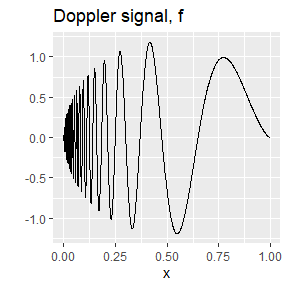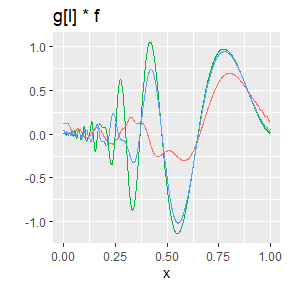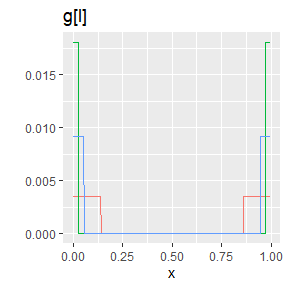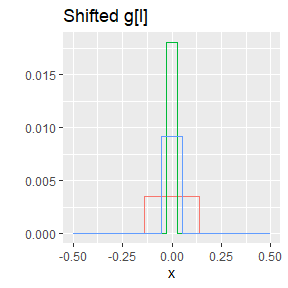## Dependence

The level of dependence is controlled by the alpha parameter mentioned in the last paragraph of the background section. The user can specify the level of dependence in each channel ($$0 < \alpha_\ell < 1$$) using a numeric vector with $$m$$ elements if known or use their own plug-in estimates of choice (the mwaved package does not estimate the dependence levels in the noise process at this stage). If the user doesn’t specify these values then the default sets each dependence level to $$\alpha_\ell = 1$$ for all $$\ell = 1, 2, \ldots, m$$ which corresponds to the case of independent error variables. Alternatively, the user can specify a single value of $$\alpha$$ and that value will be repeated across all channels. Examples of this are shown in the estimation section.

Alternative specifications: There is no universal measure of dependence. The measure that is used here is $$\alpha$$ which will take a value between 0 and 1. A value of 1 denotes the case that each noise variables is independent of the others and the long memory (long-range dependent) case occurs when $$\alpha$$ is between 0 and 1 and the level of dependence increasing as $$\alpha$$ approaches 0. The alternative specifications that might appears in the literature are in terms of the Hurst parameter $$H$$ or another dependence parameter denoted $$d$$ here,

• Hurst parametrisation: $$H = 1 - \tfrac{\alpha}{2}$$, ($$H = \tfrac{1}{2}$$ independent case).
• Dependence parameterisation: $$d = \frac{1 - \alpha}{2}$$ ($$d = 0$$, independent case).

## Simulation functions

There are many functions commonly used as examples in the literature available in the mwaved package. This package has some simulation helper functions for signals, blur and noise. The signal helper functions are covered in signals section, blur matrix generation functions in the Blur section and multichannel noise generation covered in the Noise section.

### Signal

The signal generation functions have the naming convention of the signal name with a make prefix. These examples are, makeLIDAR, makeDoppler, makeBlocks, makeBumps, makeHeaviSine and makeCusp. Below shows some code to produce the Doppler signal with plots showing a selection of four of the signals.

library(mwaved)
n <- 1024
x <- seq(from = 0, to = 1 - 1/n, length = n)
Doppler <- makeDoppler(n)
plot(x, Doppler, type = 'l')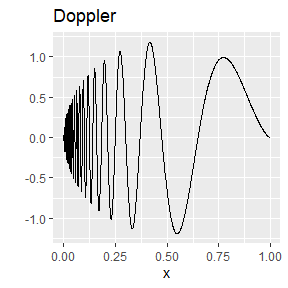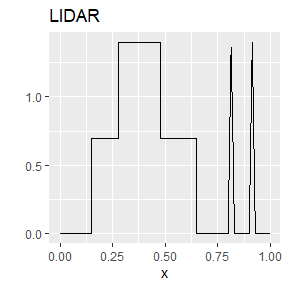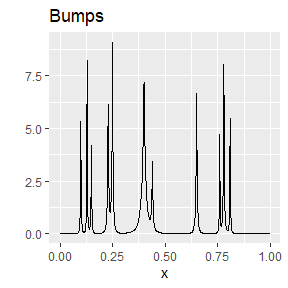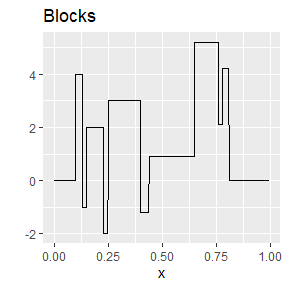### Blur

The blur generation functions have the naming convention of the density or class name followed by a Blur suffix. These examples are, gammaBlur, directBlur and boxcarBlur. The gammaBlur function produces blur of regular smooth type and is a wrapper around the dgamma function to produce appropriately scaled Gamma densities. While directBlur and boxcarBlur create blur matrices where each column is of the direct or box.car type respectively. Below shows some examples of each of the three classes with the plots on the left hand side in the time domain, $$g_j(x)$$ while the right hand side shows the energy in the Fourier domain, $$|g_{\omega, \ell}|$$.

library(mwaved)
n <- 1024
m <- 3
Gbox <- boxcarBlur(n, width = 1/sqrt(c(13, 343, 89)))
Gdir <- directBlur(n, m)
Gsmooth <- gammaBlur(n, shape = c(0.25, 0.5, 0.75), scale = rep(0.25, m))
x <- seq(from = 0, to = 1 - 1/n, length = n)

n2 <- floor(n/2)
w = -(n2 - 1):n2
Gdirfft <- mvfft(Gdir)
Gboxfft <- mvfft(Gbox)
Gsmoothfft <- mvfft(Gsmooth)
shift <- function(x) {
x <- as.matrix(x)
n2 <- dim(x)/2 + 1
y <- rbind(x[(n2+1):n,], x[1:n2,])
y
}
xlims = c(-100,100)
matplot(x, Gdir, type = 'l', main = 'Direct blur matrices')
matplot(w, shift(Mod(Gdirfft)), type = 'l', main = 'Fourier shifted', xlim = xlims)
matplot(x, Gsmooth, type = 'l', main = 'Smooth blur matrices')
matplot(w, shift(Mod(Gsmoothfft)), type = 'l', main = 'Fourier shifted', xlim = xlims)
matplot(x, Gbox, type = 'l', main = 'Boxcar blur matrices')
matplot(w, shift(Mod(Gboxfft)), type = 'l', main = 'Fourier shifted', xlim = xlims)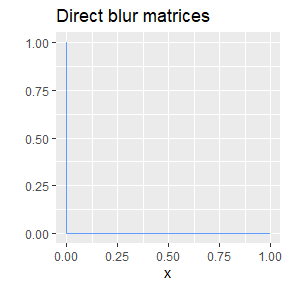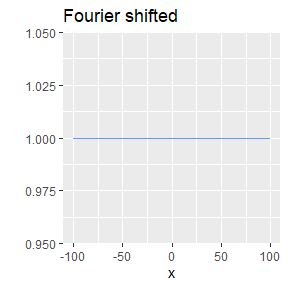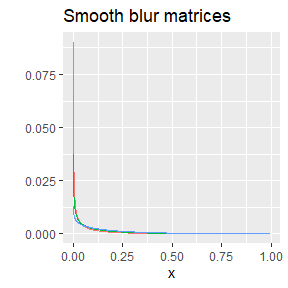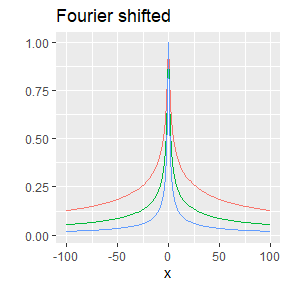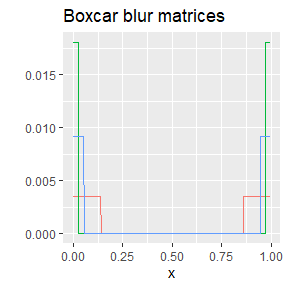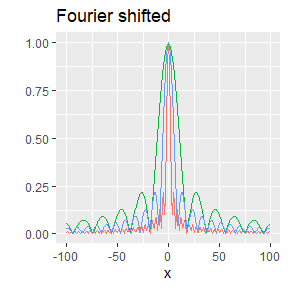### Noise

The user can also simulate multichannel noise variables, $$e_{i,\ell}$$, via the use of a wrapper function, multiNoise, that uses the fracdiff.sim function from the suggested fracdiff package or simulates using rnorm otherwise. For example, long memory error variables are simulated below and noise added to the previous blurred multichannel signals in the Data section to have a specific Blurred Signal to Noise Ratio (SNR) of 10, 20 and 30 dB, computed via the use of the helper function sigmaSNR.

library(mwaved)
n <- 1024
m <- 3
alpha <- seq(from = 0.5, to = 1, length = m)
x <- seq(from = 0, to = 1 - 1/n, length = n)
sigma <- c(1, 0.75, 0.5)
E <- multiNoise(n, sigma = sigma, alpha = alpha)
matplot(x, E, type = 'l', main = 'Long Memory Noise')
DE <- multiNoise(n, sigma = sigmaSNR(signal = Xbox, SNR = c(10, 20 ,30)), alpha = alpha)
Y <- Xbox + DE
matplot(x, Y, type = 'l', main = 'Y{i,l] = g[j]*f + e[i,l]')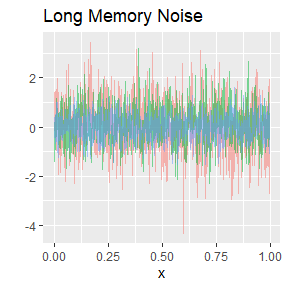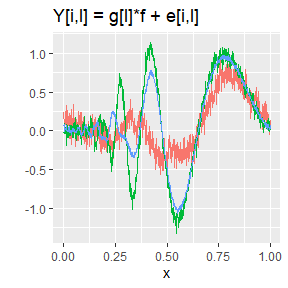# Estimation

The wavelet estimation technique is based on the inhomogeneous wavelet expansion of $$f(x)$$ which states that for any (0,1) periodic function $$f \in \mathscr L_2$$, $f(x) = \sum_{k = 0}^{2^{j_0} - 1} a_{j_0,k} \phi_{j_0,k} (x)+ \sum_{j = j_0}^{\infty} \sum_{k = 0}^{2^j-1} b_{j,k} \psi_{j,k}(x)$ where $$\left( \phi, \psi \right)$$ denotes the periodised Meyer father and mother wavelet basis functions and $$\psi_{j,k}$$ denotes the dyadic dilated and shifted functions $$\psi_{j,k}(\cdot) = 2^{j/2}\psi(2^j\cdot - k)$$ at resolution levels, $$j$$ and location $$k$$ (similar definition for $$\phi_{j_0,k}$$). While $$b_{j,k}$$ are the wavelet coefficients of $$f$$, $b_{j,k} = \int_0^1 f(x) \psi_{j,k}(x) \, dx$ ($$a_{j_0,k}$$ has a similar inner product definition involving $$\phi_{j_0,k}$$)

By changing to the Fourier domain and exploiting Parseval’s identity the wavelet coefficients can be computed with, $b_{j,k} = \int_0^1 f(x) \psi_{j,k}(x)\, dx = \sum_{\omega \in \mathbb{Z}} f_\omega \overline{ \psi_\omega^{j,k}}$ where $$\psi_\omega^{j,k}$$ denotes the Fourier transform of the Meyer wavelet basis at resolution $$j$$ and location $$k$$ with Fourier number $$\omega$$ (and $$\overline{z}$$ denotes the complex conjugate of $$z$$). The Meyer wavelet basis is bandlimited meaning that $$\psi_\omega^{j,k}$$ has a compact domain which is denoted $$C_j$$ and the cardinality $$|C_j| = 2^{j+1}$$ and the sums in the Fourier domain are finite.

Therefore, converting to the Fourier domain of the observations $$Y_{i,\ell}$$ by the FFT operator we have, $y_{\omega, \ell} = g_{\omega, \ell} f_\omega + Z_{\omega,\ell}.$

Then an estimate of the wavelet coefficients is possible by taking a weighted average of all the available channels with, $\widehat b_{j,k} = \sum_{\omega \in C_j}\frac{\sum_{\ell = 1}^m \gamma_{\omega, \ell}\overline{g_{\omega, \ell}} y_{\omega, \ell} }{\sum_{\ell = 1}^m \gamma_{\omega, \ell} |g_{\omega,\ell}|^2} \overline{\psi_\omega^{j,k}} = b_{j,k} + E_{j,k}$ The weights take the form, $$\gamma_{\omega,\ell} = n^{\alpha}\widehat \sigma_\ell^{-2}|\omega|^{1 - \alpha_\ell}$$. This weighted average has shown to be somewhat optimal as it obtains upper bounds on the risk as detailed in (Kulik, Sapatinas, and Wishart 2014). To obtain a reliable estimate the error process $$E_{j,k}$$ in the above needs to be controlled which is typically done via thresholding the coefficients.

Further, the complete inhomogeneous expansion at all resolution levels is not possible due to the deconvolution problem inflating variance of the noise variables. This requires that expansion must be truncated at an appropriate level $$j_1$$ which is estimated and the details of which are covered in the Finest resolution selection section.

Then an estimate of $$f$$ is produced by the thresholding of the estimated coefficients, $$\widehat b_{j,k}$$, at the appropriate truncation finest resolution with, $\widehat f(x) = \sum_{j = j_0}^{\widehat j_1} \sum_{k = 0}^{2^j-1} \delta_{T_j}(\widehat b_{j,k}) \psi_{j,k}(x)$ where the thresholding functions $$\delta_{T_j}$$ are defined in Thresholding section and the estimation of the coefficients $$\widehat b_{j,k}$$ are covered in the Wavelet Coefficient section. Note, the mWaveD estimate actually uses the Translation Invariant wavelet coefficients that averages over all possible shifts which was first introduced by (Donoho and Raimondo 2004). The estimated signal depends on which location the periodic function is started. Thus, for some $$h > 0$$, introduce the shift operator $$T_h f(x) = f(x + h)$$ (and similarly, $$T_{-h}f(x) = f(x - h)$$. Then define the shifted estimator $$\tilde f_h$$, that is, the wavelet estimator computed using a shifted signal $$T_h f(x)$$ instead of the base signal $$f(x)$$. Then average over the set of all possible $$n$$ shifts along the grid, $$H_n = \left\{\tfrac{1}{n}, \tfrac{2}{n}, \ldots, 1 - \tfrac{1}{n}\right\}$$ with, $\tilde f_{TI}(x) = \frac{1}{n} \sum_{h \in H_n } T_{-h}\tilde f_h(x).$ The estimator $$\tilde f_{TI}$$ is known as the Translation Invariant (TI) estimate for $$f$$ since it averages over all possible shifts and has observed to obtain a more reliable estimate. This is demonstrated in the Wavelet Coefficient section that compares the usual mWaveD estimator which is the TI estimator with the ordinary wavelet estimate that computes the wavelet coefficients of the ordinary signal and then projects them into the expansion without any circulant shifts.

The resolution level thresholds $$T_j$$ for the hard thresholding regime have been determined in (Kulik, Sapatinas, and Wishart 2014) to obtain upper bounds on the $$\mathscr L_p$$ risk and those threshold levels are used in the mwaved package. These threshold levels can be computed using either the general multiWaveD function considered below or by using the multiThresh function considered in the Wavelet Coefficient section.

There are two main estimation functions for the signal $$f(x)$$, multiEstimate and multiWaveD. The former is a function that just returns the raw wavelet deconvolution signal estimate, $$\widehat f(x)$$, while the latter returns an mWaveD object with all the necessary analysis output. In the following, a simple simulated example using the HeaviSine function will be examined. The simualted multichannel model will have three channels of which there is a good, medium and bad channel. A channel is ‘good’ in the sense of a low dependence level in the noise and high signal to noise ratio and low severity of blur in the channel. Starting with just the raw estimate function, multiEstimate, the example below shows the minimal input arguments required, the user can specify additional arguments if they wish to modify the defaults (e.g. change smoothing parameters, threshold levels or truncation level). Also, note the alpha vector need not be input if the data/model is assumed to have independent noise with no dependence structure.

library(mwaved)
n <- 1024
m <- 3
alpha <- c(0.95, 0.8, 0.75)
SNR <- c(30, 28, 25)
signal <- makeHeaviSine(n)
Gsmooth <- gammaBlur(n, shape = c(0.5, 0.66, 0.75), scale = rep(0.25, 3))
# G <- boxcarBlur(n)
X <- blurSignal(signal, Gsmooth)
E <- multiNoise(n, sigma = sigmaSNR(X, SNR))
Ysmooth <- X + E
x <- seq(from = 0, to = 1 - 1/n, length = n)
signalEstimate <- multiEstimate(Ysmooth, Gsmooth, alpha)
head(signalEstimate)
##  0.05054403 0.09966290 0.14874779 0.19779018 0.24678161 0.29571375
matplot(x, Ysmooth, type = 'l')
plot(x, signal, type = 'l')
lines(x, signalEstimate, col = 2)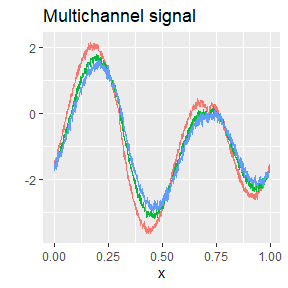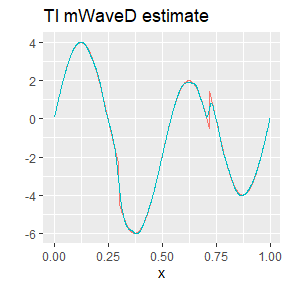Consider now the full analysis using the multiWaveD function which returns an mWaveD object which is a list with the following elements,

• signal : The noisy and blurred multichannel signal, $$Y_{i,\ell}$$ that the user input in the form of a numeric $$n \times m$$ matrix (see Data).
• G : The ‘blur’ matrix $$G$$ whose $$\ell^{\rm th}$$ column is given by, $$g_{\ell,n}[i]$$, that the user input in the form of a numeric $$n \times m$$ matrix (see Data).
• j0 : The coarsest resolution level in the wavelet expansion, $$j_0$$, either user specified or starting at the default, $$j_0 = 3$$.
• j1 : The estimated finest resolution level for the wavelet expansion, $$\widehat j_1$$, either user specified or estimated using one of two possible resolution selection methods covered in the Finest resolution selection method section.
• resolutionMethod : The resolution selection method used to estimate $$j_1$$, either ‘smooth’ or ‘block’ to refer to the methods covered in Smooth resolution selection or Blockwise variance selection sections respectively.
• blurDetected : The detected form of the blur matrix, ‘G’, that corresponds to the $$g_\ell(x_i)$$ kernel. Simple method identifies if the matrix appears to be of direct class or box.car class or returns smooth otherwise.
• alpha : The vector that specifies the dependence level in each channel following the parametrisation given in Dependence
• sigmaEst : The estimate of the noise scale levels in each channel (standard deviation of the noise, $$\widehat \sigma_\ell$$ an estimate of $$( \mathbb{E} e_{i,\ell}^2 )^{1/2}$$. This is estimated by computing the Median Absolute Deviation of the wavelet coefficients in the highest possible resolution level.
• blurInfo : The output created in the resolution selection method analysis. Either contains a list with Fourier energy levels and their associated bounds in the smooth method or a list with computed variance levels and their bounds in the block method.
• eta : The smoothing parameter used in the thresholding of the wavelet coefficients.
• thresholds : The resolution level thresholds, $$T_j$$, that were applied to the raw wavelet coefficients, $$\widehat b_{j,k}$$.
• estimate : The translation invariant wavelet deconvolution estimate of $$f$$.
• coef : The raw wavelet coefficients, $$\widehat b_{j,k}$$
• shrinkCoef : The thresholded estimated wavelet coefficients, $$\delta_{T_j}(\widehat b_{j,k})$$
• percent : The percentage of coefficients that are thresholded in each resolution level used in the expansion. That is, the percentage where $$|\delta_{T_j}(\widehat b_{j,k})| < |\widehat b_{j,k}|$$.
• levelMax : The largest wavelet coefficient in each resolution level.
• shrinkType : The type of shrinkage used in the thresholding of the wavelet coefficients, either ‘hard’, ‘soft’ or ‘garrote’.
• projections : The wavelet projections at each resolution level. Namely, for resolution $$j$$, each column corresponds has $$i^{\rm th}$$ element given by $$\sum_{k = 0}^{2^j-1} \delta_{T_j}(\widehat b_{j,k})\psi_{j,k} (x_i)$$, for $$i = 1, 2,\ldots, n$$.
• noise : The estimated noise process in each channel.
• degree : Degree of the auxiliary Meyer polynomial used in the Meyer wavelet.
signalmWaveD <- multiWaveD(Ysmooth, Gsmooth, alpha)
names(signalmWaveD)
##   "signal"           "G"                "j0"
##   "j1"               "resolutionMethod" "blurDetected"
##   "alpha"            "sigmaEst"         "blurInfo"
##  "eta"              "thresholds"       "estimate"
##  "coef"             "shrinkCoef"       "percent"
##  "levelMax"         "shrinkType"       "projections"
##  "noise"            "degree"
plot(signalmWaveD)
## Warning: Using size for a discrete variable is not advised.
## Warning: Using size for a discrete variable is not advised.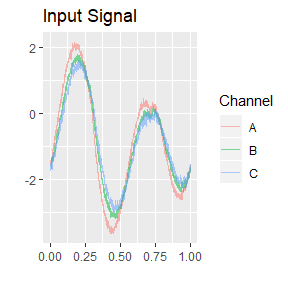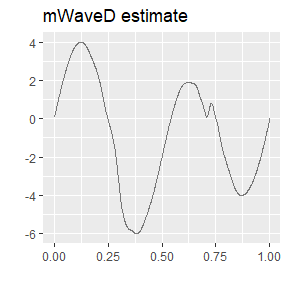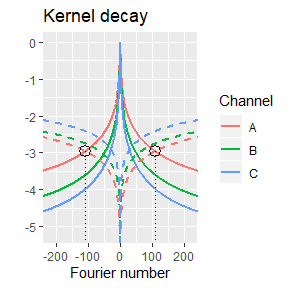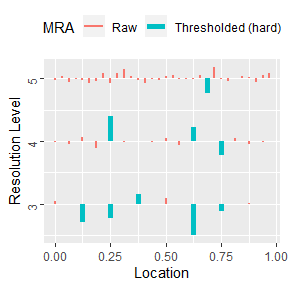The default plot method shows 4 plots in the above 2 by 2 grid. The user can decide to plot only a specific single plot if they wish by using an additional argument. E.g. if you just want the Multiresolution analysis plot, specify the 4th plot with:

plot(signalmWaveD, which = 4)
## Warning: Using size for a discrete variable is not advised.A short analysis is possible by looking at the summary of the R mWaveD object. This succinctly describes the mWaveD analysis using elements listed in the mWaveD object.

summary(signalmWaveD)
## Degree of Meyer wavelet = 3   , Coarse resolution level j0 = 3
## Sample size per channel = 1024, Maximum possible resolution level = 9.
##
## Number of channels: m = 3
## Detected Blur Type: smooth
##
## Resolution selection method:  smooth
##
## Estimated Channel information:
##
##            Sigma.hat Alpha Fourier number cutoff Highest resolution
## Channel 1:     0.060  0.95                   110                  5
## Channel 2:     0.062  0.80                    53                  4
## Channel 3:     0.084  0.75                    30                  3
##
## Estimated best channel = Channel 1
##
## mWaveD optimal finest resolution level j1 = 5
##
## Thresholding method: hard    Tuning parameter: eta = 3.898718
##
##           Max|w| Threshold % Shrinkage
## Level 3 : 0.1387    0.0286      37.500
## Level 4 : 0.1136    0.0426      81.250
## Level 5 : 0.0678    0.0646      96.875

## Finest resolution selection

The claim was made in the Estimation section that there is a problem with using higher resolutions in the wavelet estimate since the noise gets inflated in the Fourier deconvolution paradigm. Indeed, consider the weighted averaging of the Fourier wavelet coefficient estimates, $\widehat b_{j,k} - b_{j,k} = E_{j,k} = \sum_{\omega \in C_j} \frac{\sum_\ell \gamma_{\omega,\ell} \overline g_{\omega,\ell} E_{\omega,\ell} \overline{\psi_{\omega}^{j,k}}}{\sum_\ell \gamma_{\omega,\ell} |g_{\omega,\ell}|^2}.$ where $$E_{\omega, \ell}$$ denotes the FFT of the original model noise variables (FFT applied over each column).

In the Fourier domain, for the indirect cases, $$g_{\omega,\ell}$$ will decay as $$|\omega| \to \infty$$ and in particular, division by the decaying $$|g_{\omega, \ell}|^2$$ causes instabilities by inflating the noise variables $$E_{\omega, \ell}$$. This noise inflation can dominate the desired wavelet coefficient signal $$b_{j,k}$$ embedded in side the empirical estimate $$\widehat b_{j,k}$$.

Optimal stopping points for the resolution parameter, $$j$$, the finest resolution level, have been determined in (Kulik, Sapatinas, and Wishart 2014). For the regular smooth case, the optimal level is at, $2^{j_1} \asymp \left( \frac{n^{\alpha_{\ell_*}}}{\log n}\right)^{1/(2\nu_{\ell_*} + \alpha_{\ell_*})}$ where $$\ell_*$$ is the channel that minimises the variance of the coefficients is determined as, $\ell_* := \text{arg min}_{1 \le \ell \le M} n^{-\alpha_\ell}2^{\alpha_\ell + 2\nu_\ell}.$ For the box.car case, the optimal finest resolution level is, $2^{j_1} \asymp \left( \frac{n^{\alpha_*}}{\log n}\right)^{1/(2\alpha_* + 1 + 1/m))}$ where $$\alpha_* = \min_{1 \le \ell \le m} \alpha_\ell$$.

However, these are asymptotic conditions and a numerical method is required for the user that will adapt to the data. Therefore two numerical finest resolution selection methods that are presented in the mWaveD package, the smooth method and the block method.

As the name suggests, the smooth method is the recommended technique for the regular smooth class of convolving functions. It is however, not recommended for the box.car class since the box car functions are very erratic in the Fourier domain (see for example the plots in the Data section) and the method truncates too early as is seen in the upcoming section.

### Smooth resolution selection method

The recommendation for the smooth class is backed up by the theory presented in (Kulik, Sapatinas, and Wishart 2014) and (Wishart 2013), which itself is based on the results in [@CR2007. The interested reader is referred there for precise details. In particular, it is shown that $$\widehat j_1$$ will be close to $$j_1^{[S]}$$ with high probability using this method.

A rough intuitive argument will be presented here for the reader. As determined by the theory, the best available channel will determine the truncation level, $$j_1$$, when the convolving functions are of regular smooth class. With this in mind, the estimate $$\widehat j_1$$ is then estimated by looking at the decay of the convolving functions piecewise for each channel and taking the best performer. For ease of intuition, consider the single channel case with regular smooth blur where the noise process takes the form,

$E_{j,k} = \sum_{\omega \in C_j} \frac{E_{\omega, \ell} \overline{\psi_\omega^{j,k}}}{g_{\omega, \ell}} \asymp \sum_{\omega \in C_j} |\omega|^{\nu}E_{\omega, \ell} \overline{\psi_\omega^{j,k}}$

Intuitively, a bound is found for when the maximum of the inflated noise variables will dominate the decaying $$|g_{\omega,\ell}|$$ and knowledge required for inversion is lost and becomes unstable.

This can be controlled in the mWaveD estimates by specifying resolution = 'smooth' as an argument in either the multiWaveD function or the multiEstimate function. This will force the smooth resolution selection method to be used. If the box.car blur is detected and the user has specified resolution = 'smooth', then a warning will be thrown to the user that this is an unreliable method as the plots below show which is based on the earlier simulations.

The plots below show this process using a log scale on the vertical axis. Namely, the solid line corresponds to $$\log |g_{\ell}|$$ and the vertical line the calibrated bound.

smoothObj <- multiWaveD(Ysmooth, Gsmooth, alpha, resolution = 'smooth')
plot(smoothObj, which = 2)
plot(smoothObj, which = 3)

Xbox <- blurSignal(signal, Gbox)
E <- multiNoise(n, sigma = sigmaSNR(Xbox, SNR))
Ybox <- Xbox + E

boxObjSmooth <- multiWaveD(Ybox, Gbox, alpha = 1, resolution = 'smooth')
## Warning in blurCheck(G, resolution): resolution = "smooth" specified but input G appears to be box.car.
##  Danger of early truncation and j1 being too small, suggest change resolution selection method to "block".
bsj1 <- boxObjSmooth$j1 boxObjBlock <- multiWaveD(Ybox, Gbox, alpha = 1, resolution = 'block') bbj1 <- boxObjBlock$j1
paste0('estimate j1 = ',bsj1, ' or ',bbj1, ' for the smooth and block method respectively.')
##  "estimate j1 = 3 or 5 for the smooth and block method respectively."
plot(boxObjSmooth, which = 2)
plot(boxObjSmooth, which = 3)
plot(boxObjBlock, which = 2)
plot(boxObjBlock, which = 3)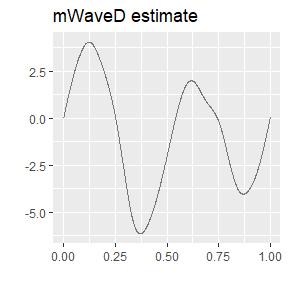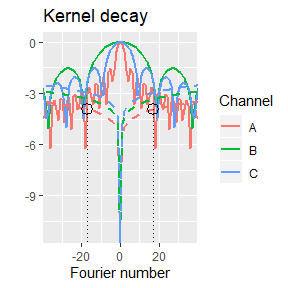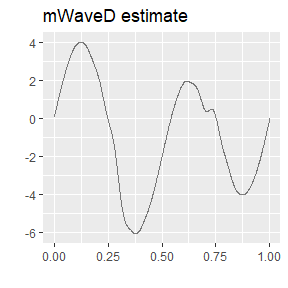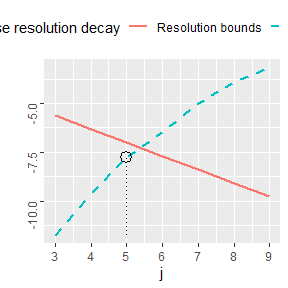Notice that when smooth blur is used, the smooth resolution method works well and the estimated truncation levels allows the estimate to capture some information from the discontinuity.

On the other hand, when box.car blur is used, the smooth method truncates too early, it estimates the finest resolution at $$\widehat j_1 = 3$$. The resulting wavelet expansion doesn’t capture the high resolution behaviour at the discontinuity. The block method does truncate appropriately at $$\widehat j_1 = 5$$ instead and captures the discontinuity in the presence of box.car blur.

As a final remark, this is the selection method used in the waved package and is actually applicable for the case when the user does not have knowledge of $$g_\ell$$ but instead only within noise. That is, the user only knowledge of $$k_{\ell,n}[i]$$ where, $k_{\ell,n}[i] = g_{\ell,n}[i] + \epsilon_i$ where $$\epsilon_i$$ are a set of noise variables.

The method is still applicable and could be used, since it will still estimate finest resolution level $$\widehat j_1$$ appropriately. For example,

NGsmooth <- Gsmooth + multiNoise(n, sigma = sigmaSNR(Gsmooth, rep(10,3)))
noisySmoothObj <- multiWaveD(Ysmooth, NGsmooth, alpha, resolution = 'smooth')
plot(smoothObj, which = 3)
plot(noisySmoothObj, which = 3)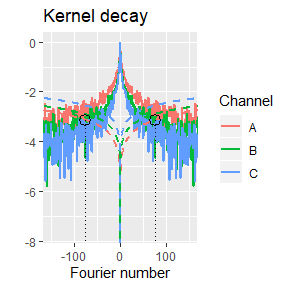As expected, earlier truncation occurs in the case of noisy blur. For inversion, the noisy blur is more numerically unstable than the noiseless blur information. This is seen since the Fourier numbers are 76 and 110 respectively for the noisy and noiseless blur information cases.

However, performance of the general wavelet estimate is not guaranteed since thresholds for denoising are not calibrated for this case and no theoretical results for this case are available at this stage.

### Blockwise variance resolution selection method

The block resolution selection method is based on (Cavalier and Raimondo 2006), (Wishart 2014) and the result in (Johnstone et al. 2004). The box.car class of blur functions are erratic in the Fourier domain as seen in the Data section. This instabilities can be avoided by summing over dyadic blocks as shown by (Johnstone et al. 2004), $\sum_{\omega \in C_j} 2^{-j} |g_{\omega, \ell}|^{-2} \asymp 2^{3j/2}.$ This fact is combined with the variance of th empirical wavelet coefficient estimates at each resolution which are of the form, $\mathbb{V}\text{ar} \left( \widehat b_{j,k}\right) = \sum_{\omega \in C_j} |\psi_\omega^{j,k}|^2 \sigma_\ell^2/n^{\alpha_\ell}|\omega|^{\alpha_\ell - 1}|g_{\omega,\ell}|^{-2}$ Then the blockwise resolution selection method is a similar stopping rule that checks whether the variance of the empirical coefficients at each resolution (block) exceeds a threshold that agrees with the optimal level $$j_1$$. The resolution at which the block variance exceeds the threshold is deemed unusable and the previous resolution is the estimated finest resolution $$\widehat j_1$$. Again, a log scale is used on the vertical axis, since the variance grows at a rate $$2^j$$, meaning the log scaled version is linear in $$j$$.

plot(boxObjBlock, which = 2)
plot(boxObjBlock, which = 3)## Thresholding

There are three thresholding types available in mwaved, all of which are resolution level thresholding regimes and require a single threshold parameter $$T$$ for each resolution level in the Wavelet expansion. These are the ‘hard’, ‘soft’ and ‘garrote’ regimes. These thresholding functions are of the form, $$\delta = \delta_T(x)$$

• Hard : $$\delta_T(x) = 1_{\left\{ |x| \ge T\right\}}x$$
• Soft : $$\delta_T(x) = sign(x)1_{\left\{ |x| \ge T\right\}}\left( |x| - T \right)$$
• Garrote : $$\delta_T(x) = 1_{\left\{ |x| \ge T\right\}}\left(x - \frac{T^2}{x}\right)$$

The examples below show the three thresholding regimes over the domain $$x \in (-3,3)$$ and threshold parameter $$T = 1$$.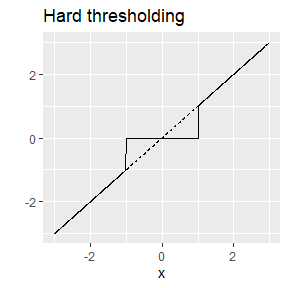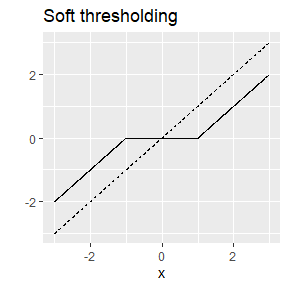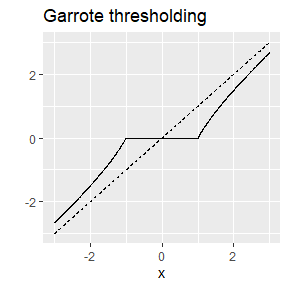## Wavelet Coefficients

Computing the wavelet coefficients of $$f$$ using the noisy blurred signal is done in the Fourier domain by the previously presented method in the initial Estimation section. This can also be focussed on separately using the multiCoef function. This function creates a waveletCoef object that is a list containing the following elements.

wave <- multiCoef(Ysmooth, Gsmooth, alpha = alpha)
str(wave)
## List of 3
##  $coef: num [1:1024] 0.0114 1.4248 -0.0919 -2.1422 -0.6491 ... ##$ j0  : int 3
##  \$ deg : int 3
##  - attr(*, "class")= chr "waveletCoef"
names(wave)
##  "coef" "j0"   "deg"

Given an inhomogeneous wavelet expansion is desired starting at resolution $$j_0$$, the structure of the waveletCoef object is list with the following elements,

• coef: Numeric vector of wavelet coefficients, the first $$j_0$$ values are the coarse wavelet coefficients for the father wavelet part of the expansion involving $$\phi_{j_0,k}$$. The remaining coefficients are the detail coefficients of the expansion involving the $$\psi_{j,k}$$ for $$j = j_0, \ldots, \widehat j_1$$.
• j0: The coarse resolution used in the computation of the wavelet coefficients, default j0 = 3.
• deg: The degree of the auxiliary polynomial used in the Meyer wavelet, default deg = 3.

### Plotting coefficients (MRA)

There is a plot method available for the waveletCoef object that shows a multiresolution analysis. This plot method can take a single waveletCoef object as an x argument or two waveletCoef objects as x and y arguments. See for example below, the wavelet coefficients of the LIDAR signal and a noisy version.

library(mwaved)
n <- 1024
signal <- makeLIDAR(n)
Y <- signal + multiNoise(n, sigma = sigmaSNR(signal, 20))
# Without thresholding
rawWave <- multiCoef(Y)
thresh <- multiThresh(Y)
# With thresholding
threshWave <- waveletThresh(rawWave, thresh)
sigCoefs <- multiCoef(signal)
plot(sigCoefs)
plot(rawWave, threshWave)
## Warning: Using size for a discrete variable is not advised.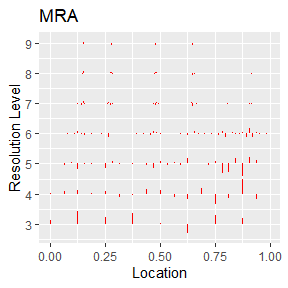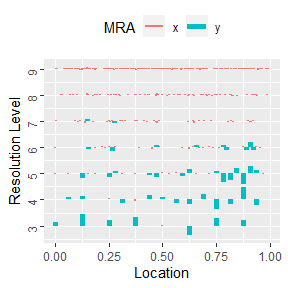### Projection of coefficients

You can also take a set of wavelet coefficients and project the wavelet expansion using the multiProj function. Using the computed coefficients from the MRA section,

x <- seq(from = 0, to = 1 - 1/n, length = n)
plot(x, multiProj(sigCoefs), ty = 'l', main = 'signal coefs')
plot(x, multiProj(rawWave), ty = 'l', main = 'noisy signal coefs')
plot(x, multiProj(threshWave), ty = 'l', main = 'thresholded signal coefs')
plot(x, multiEstimate(Y), ty = 'l', main = 'TI mWaveD estimate')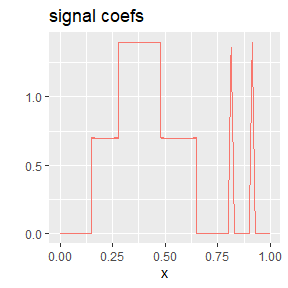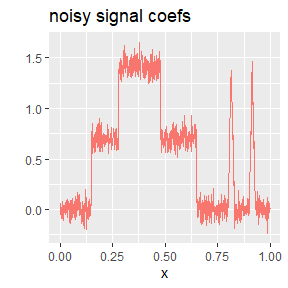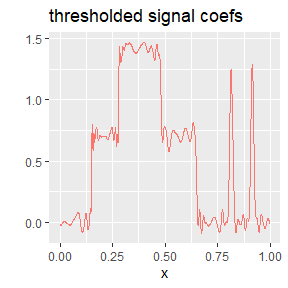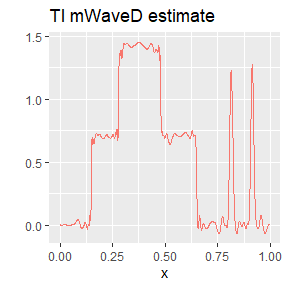Note the difference between the projected thresholded coefficients which would be the standard wavelet estimate and the TI mWaveD estimate with the TI estimate appearing to estimate the signal more accurately.

## Noise scale estimation

The wavelet coefficients can also be used to estimate scale of noise in each channel. That is, estimate $$\sigma_\ell = \sqrt{\mathbb{V}\text{ar}e_{i,\ell}}$$. A standard method to do this is to consider the empirical wavelet coefficients in the highest possible resolution level. The idea being that the noise variables are the most dominant in the highest resolution and the signal least dominant. So, for a signal of length $$n$$. The last possible resolution level is at, $j_{\max} = \lfloor \log_2 n \rfloor - 1.$ Then compute the empirical wavelet coefficients at the highest possible level for data in each channel, denoted with say, $$\widehat b_{j_{\max}, k}^{[\ell]}$$ for channel $$\ell$$. Then using any scale estimator, one can obtain an estimate for $$\sigma_\ell$$. The popular robust choice is to use the Median Absolute Deviation (MAD). Therefore, separately for each channel, estimate $$\sigma_\ell$$ with, $\widehat \sigma_\ell = 1.4862 \, MAD_{k}(\widehat b_{j_{\max}, k}^{[\ell]})$ where $$1.4862$$ is the usual normalising constant for Gaussian random variables. This is exactly the method provided by the multiSigma function that is available to the user and used in the other functions, multiEstimate, multiThresh and multiWaveD when necessary. An example of simulated noise and scale estimation is provided below.

library(mwaved)
sigma <- c(0.5, 0.75, 2)
n <- 1024
E <- multiNoise(n, sigma = sigma)
multiSigma(E)
##  0.5491546 0.7904135 1.9473397

# Interactive Applet (Shiny)

If the user has the shiny R package installed, the user is recommended to explore the interactive Shiny applet. The applet allows the user to explore the capabilities of the package and returns the base commands to produce the output seen in the applet. It can be run locally on their system by loading mwaved package and launching the applet with,

library(shiny)
library(mwaved)
mWaveDDemo()